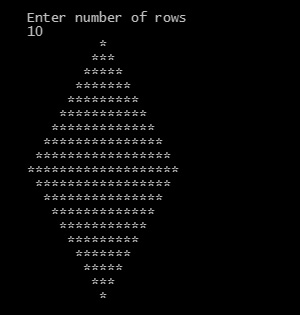# C Program to Print Diamond Pattern

This C code print stars, which makes a diamond pattern.

## Create diamond pattern in C by using nested for loop

Program:
``````#include <stdio.h>

int main()
{
int n, c, k, space = 1;

printf("Enter number of rows\n");
scanf("%d", &n);

space = n - 1;

for (k = 1; k <= n; k++)
{

for (c = 1; c <= space; c++)
printf(" ");

space--;

for (c = 1; c <= 2*k-1; c++)
printf("*");

printf("\n");

}
space = 1;

for (k = 1; k <= n - 1; k++)
{

for (c = 1; c <= space; c++)
printf(" ");

space++;

for (c = 1 ; c <= 2*(n-k)-1; c++)
printf("*");

printf("\n");

}

return 0;
}
``````
Output of program:Explanation:

This program is used to make the diamond pattern using asterisk symbol. So first of all, you have to include the stdio header file using the "include" preceding # which tells that the header file needs to be process before compilation, hence named preprocessor directive.

Then you have to define the main() function and it has been declared as an integer so by default it returns an integer. Inside the main() function you have to declare four integer type variable name - 'n', 'c', 'k', 'space' and initialize the value of space as '1'. Then a printf() function is used which will print the message - "Enter number of rows". The scanf() function ta=hen takes the value from the user and puts in variable 'n'. Then the value of space gets initialized to space = n-1.

Now, a for loop will be required which will start from k=1  to k<=n. Then another nested for loop will be used which will go from c=1 till c<=space, and using post-decrement operator, decrements the value of space.

Another nested for loop needs to be implemented which goes from c=1 till c<= 2*k-1. And within that nested for loop the printf() isused to display the asterisk (*) symbol. The next printf() is used to print next line. The return 0; statement is used to return an integer type value back to main().